# Polynomial Time Trend with Python

• October 01, 2020 |

Get the data on Github if you don't have it already. You will also need to go back to get the BacktestSA from here if you don't have it yet, along with the DataManager class.

In this strategy we will create a rolling polynomial time trend, which will spit out a prediction on the price for our predefined holding period. The OLS model is as follows with $$t$$ being a time component:

$$y = X\beta + \epsilon$$

Where $$y$$  is the vector of observations, in our case the price of Bitcoin/Ethereum. $$\beta$$  is a vector of coefficients. The matrix $$X$$ takes the form shown below:

$$X = \begin{bmatrix} 1 & t_{1} & t_{1}^2 \\ 1 & t_{2} & t_{2}^2 \\ \vdots & \vdots &\vdots \\ 1 & t_{m} & t_{m}^2 \end{bmatrix} , \ \ \ X^T = \begin{bmatrix} 1 & 1 &\cdots& 1 \\ t_{1} & t_{2} &\cdots &t_{m}\\ & \\ t_{1}^2&t_{2}^2& \cdots &t_{m}^2\end{bmatrix}, y=\begin{bmatrix} y_{1}\\ y_{2}\\\vdots\\y_{m}\end{bmatrix}, \beta = \begin{bmatrix} \beta_{0} \\\beta_{1}\\\beta_{2}\end{bmatrix}$$

Minimize the sum of squared errors to solve for $$\beta$$:

$$\beta = \underset{\beta}{\arg\min}||y - X\beta||_{2}^{2}$$

Expanding the function above and taking first derivative, w.r.t $$\beta$$ , setting to zero and rearranging a bit will give us the normal equation.

$$\beta = (X^TX)^{-1}X^{T}y$$

One could argue the following is a more intuitive way to look at the above equations. Let's take an example with only 5 observations, and then put some numbers to the formulae above.

$$\begin{bmatrix} y_{1}\\ y_{2}\\y_{3}\\y_{4}\\y_{5} \end{bmatrix} = \beta_{0} \begin{bmatrix} 1 \\ 1\\1\\1\\1 \end{bmatrix} + \beta_{1} \begin{bmatrix} t_{1} \\ t_{2}\\t_{3}\\t_{4}\\t_{5} \end{bmatrix} +\beta_{2} \begin{bmatrix} t_{1}^2 \\ t_{2}^2\\t_{3}^2\\t_{4}^2\\t_{5}^2 \end{bmatrix}$$

So above we have 3 $$\beta$$ coefficients, let's look at a numerical example in which the numbers were chosen for illustrative purposes. The vector to the left of the equality sign is the price of Bitcoin in USD, the vector of ones is for the intercept, the vector 1-5 is the linear time component, and the vector being multiplied by $$\beta_{2}$$ is the square of the linear component. So all we need to do is solve for $$\beta_{0}, \beta_{1}\ and\ \beta_{2}$$

$$\begin{bmatrix} 10000\\ 10200\\10900\\11900\\13400 \end{bmatrix} = \beta_{0} \begin{bmatrix} 1 \\ 1\\1\\1\\1 \end{bmatrix} + \beta_{1} \begin{bmatrix} 0 \\ 1\\2\\3\\4 \end{bmatrix} +\beta_{2} \begin{bmatrix} 0 \\ 1\\4\\9\\16 \end{bmatrix}$$

In Python:

import numpy as np
import matplotlib.pyplot as plt
np.set_printoptions(precision=3,suppress=True)

y = np.array([10000,10200,10900,11900,13400]).reshape(-1,1)

t = np.arange(len(y))
X = np.c_[np.ones_like(y), t, t**2]

print(y)
print(X)
Out:
array([,
,
,
,
])
Out:
array([[ 1,  0,  0],
[ 1,  1,  1],
[ 1,  2,  4],
[ 1,  3,  9],
[ 1,  4, 16]])

Solving for the vector of betas using the normal equation: $$\beta = (X^TX)^{-1}X^{T}y$$

betas = np.linalg.inv(X.T@X)@X.T@y
Out:
array([[9994.29],
[  21.43],
[ 207.14]])

$$\beta_{0} = 9994.29 , \ \beta_{1} = 21.43,\ \beta_{2} = 207.14$$

We can then use the coefficients from above to make new predictions as follows:

$$\hat{y} =X\beta$$

$$\epsilon = y - \hat{y}$$

Let's make two arrays, one for the in-sample predictions, and another for the extrapolated predictions.


insample = X@betas
t_new = np.arange(9).reshape(-1,1)
X_test = np.c_[np.ones_like(t_new), t_new, t_new**2]

out_sample = X_test@betas

plt.plot(t_new, out_sample,label='Extrapolated Path',linestyle=':',linewidth=3,color='black')
plt.plot(insample ,label='Insample Fit',linewidth=3,color='red')
plt.plot(y, marker='o',linestyle="None",label='True Values',color='blue')
plt.xlabel('Periods')
plt.ylabel('Price in \$')
plt.legend(loc='best')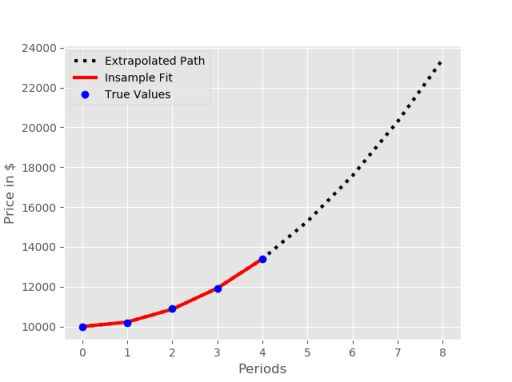Essentially we are going to select from the dashed black line, in order to get our prediction for the future. Since playing with polynomials can result in extreme/unrealistic values, it is probably best to select as small a look-ahead period as possible.

In order to try to predict the non-linear movement in the crypto futures, we will select only the most extreme predictions to try to predict and profit from. Here is where a bit of data snooping takes place: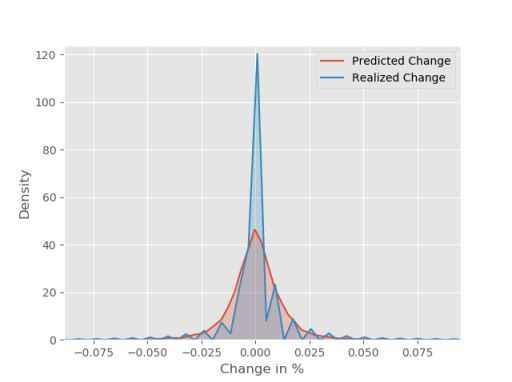The red curve, that looks remarkably like a Laplace distribution, shows the predicted change distribution versus the realized change (blue). I have decided to try to predict the tails, I have defined the cut-offs rather unscientifically as -+ 3% (For the hourly timeframe). Therefore, in the entry conditions below the long and short thresholds are 3%. We want to be able to pass in the threshold as an argument as we try different timeframes.

LONG

1 : if $$\frac{predicted}{close}-1 \geq \text Threshold \ \text long$$

SHORT

-1 : if $$\frac{predicted}{close}-1 \leq \text Threshold \ \text short$$

Up to this point, we have been using symmetric barriers for the targets/stops (here if you have not seen section on barriers). In this section, we will also add the ability to change this. So for example: for longs we can have a target price of 4% and a stop of 2% etc.

A small sample of the results shown in the video:

I decided to use a 24 hour fixed lookback window for all the timeframes shown in the video. Therefore for hourly data: lookback = 24, 30min: lookback =48 and so on.

It seems like we got some interesting results on 5min, 15 min, 30 min & 60 min timeframes. In order to save space I have only included the results from the 60min timeframe for both Ethereum and Bitcoin. See the video for more details.

Bitcoin

Even 2 % Barriers for Stops and Targets.  245 trades.

Long & Short Threshold is -+3%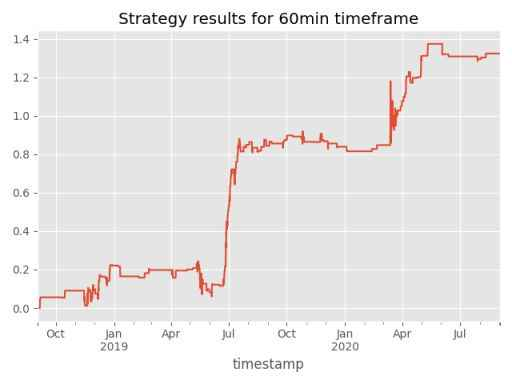Uneven Barriers: Long Target = 3%, Long Stop = 2% , Short Target = 4% , Short Stop = 2.5%

(Numbers above chosen to show that uneven barriers can be applied)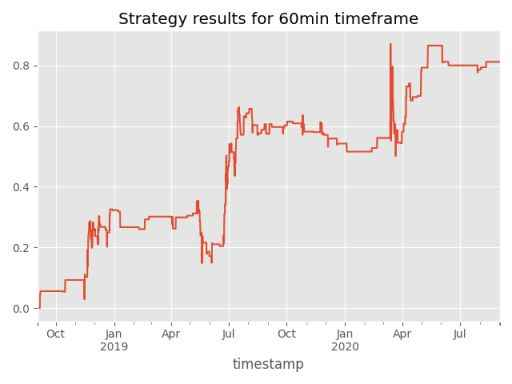It looks we we have done worse when applying the uneven barriers for targets and stops. There are a number of interesting ways you could determine these barriers. For example in Marcos Lopez De Prado's Advances in Financial Machine learning, he sets the barriers as a function of rolling volatility.

Ethereum

Even 2 % Barriers for Stops and Targets. 332 trades.

Long & Short Threshold is -+3% (Probably should have been set higher as Eth is more volatile)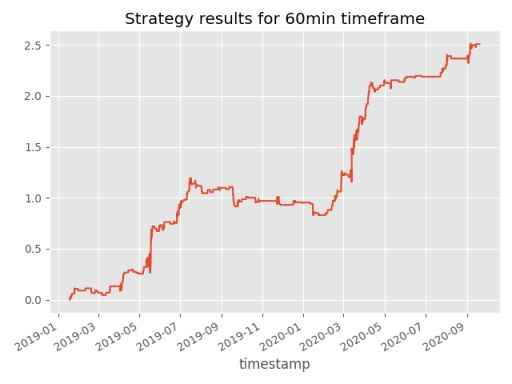Uneven Barriers: Long Target = 3%, Long Stop = 2% , Short Target = 4% , Short Stop = 2.5%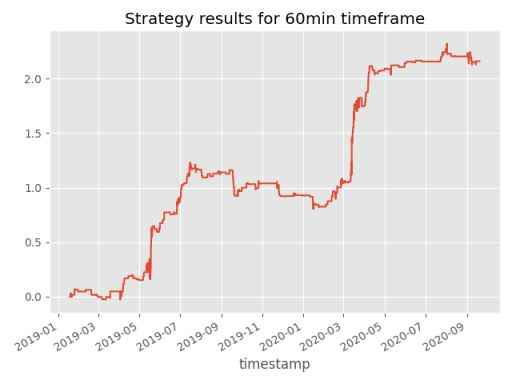Although we make some pretty unrealistic assumptions, along with neglecting transaction costs, the strategy looks quite interesting.

Code to replicate results :

from Backtesting.Backtest import BackTestSA
import pandas as pd
import numpy as np
import matplotlib.pyplot as plt

class PolyTrend(BackTestSA):

def __init__(self, csv_path, date_col, max_holding,
lookback, look_ahead, long_thres, short_thres,
ub_mult, lb_mult, long_tp, long_sl,
short_tp, short_sl):

super().__init__(csv_path, date_col, max_holding)

self.lookback = lookback
self.look_ahead = look_ahead

self.long_thres = long_thres
self.short_thres = short_thres

self.ub_mult = ub_mult
self.lb_mult = lb_mult

self.long_tp = long_tp
self.long_sl = long_sl
self.short_tp = short_tp
self.short_sl = short_sl

@staticmethod
def rolling_tt(series, n):
'''

:param series: close price for eth/btc in pandas series
:param n: lookahead from constructor
:return: prediction for close_{+n}
'''

y = series.values.reshape(-1, 1)
t = np.arange(len(y))
X = np.c_[np.ones_like(y), t, t ** 2]
betas = np.linalg.inv(X.T @ X) @ X.T @ y
new_vals = np.array([1, t[-1]+n, (t[-1]+n)**2])
pred = new_vals@betas  # beta0 + beta1 * t[-1]+n + beta2 * (t[-1]+n)**2
return pred

def generate_signals(self):
df = self.dmgt.df
n = self.look_ahead
df['preds'] = df.close.rolling(self.lookback).apply(self.rolling_tt,
args=(n,), raw=False)
#predicted change column
df['pdelta'] = (df.preds/df.close)-1
df['longs'] = (df.pdelta > self.long_thres)*1
df['shorts'] = (df.pdelta < self.short_thres)*-1
df['entry'] = df.longs + df.shorts
df.dropna(inplace=True)

def run_backtest(self):

self.generate_signals()
for row in self.dmgt.df.itertuples():
if row.entry == 1:

if self.open_pos is False:
#setting the target and stop, see BacktestSA class to see why this works
self.ub_mult = self.long_tp
self.lb_mult = self.long_sl
self.open_long(row.t_plus)
else:
self.monitor_open_positions(row.close, row.Index)
elif row.entry == -1:

if self.open_pos is False:
self.ub_mult = self.short_sl
self.lb_mult = self.short_tp
self.open_short(row.t_plus)
else:
self.monitor_open_positions(row.close, row.Index)

elif self.open_pos:
self.monitor_open_positions(row.close, row.Index)
else:
self.add_zeros()

self.add_trade_cols()

if __name__ == '__main__':
csv_path = "data/cleaned_btc.csv"
date_col = 'timestamp'
max_holding = 12*12
lookback = 12*24 # I fixed this at 24 hour minimum, 60min = 24, 30min = 48 etc
look_ahead = 4 # periods ahead to predict for each endpoint of the model
long_thres = 0.03 #if model predicts a price that represents an increase of 3% we enter
short_thres = -0.03 # opposite of above
ub_mult = 1.02
lb_mult = 0.98
long_tp = 1.03 #long target in %
long_sl = 0.98 # long stop loss
short_tp = 0.96 # short target
short_sl = 1.025 # short stop loss

Poly = PolyTrend(csv_path, date_col, max_holding,
lookback, look_ahead, long_thres,
short_thres, ub_mult, lb_mult, long_tp,
long_sl, short_tp, short_sl)

Poly.dmgt.change_resolution('5min')
Poly.run_backtest()
Poly.show_performace()
print(abs(Poly.dmgt.df.direction).sum())


Polynomial Time Trend with Python Learn to code trading algorithms for crypto in Python